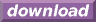#Calcute: freeware calculator

## Arcsine (arc-sine, inverse sine, asin)

The arc-sine is the inverse of the sine function. Calcute implements it with the asin function. The inverse sine of a value x is the value y for which the sine of y is x. In other words if y = asin(x) then x = sin(y). And since the sine function takes an angle as input parameter, the arcsine produces an angle as its output.

```asin(1)
1.57079632679
```

The asin value is expressed by Calcute using the currently-selected angle unit: radian, degree or gradient. In the above example, the result is in radians.

The arc-sine function is only defined in the range [-1, +1]; this matches the output range of the sine function. Note also that the sine function is periodic: its values repeat infinitely for angles that represent more than one complete rotation. Conversely, the arcsine is a multi-valued function: any parameter value matches an infinite number of possible angles. But the asin function generates a single value refered to as the principal value. All other possibilities can be obtained by adding or subtracting any whole number of rotations from the return value of asin.

In some mathematical texts and hand-held calculators, the notation sin-1 is sometimes used to represent the arc-sine function. This is an unfortunate notational choice since it could also stand for the multiplicative inverse 1/sin, which is a different function.

Yet another term also used to represent the inverse sine function is "arcsin".